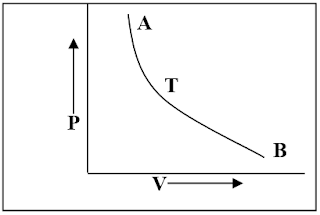Showing posts with label Heat & Thermodynamics. Show all posts
Showing posts with label Heat & Thermodynamics. Show all posts

Entropy:

The term ‘Entropy’ is a Greek word and means transformation. Entropy is a physical property or nature of a substance that remain constant in an adiabatic process. It is a quantity that increases with the application of heat in a reversible manner.

If, a process or cycle reject or absorb heat of amount dQ in T temperature, then entropy,Carnot’s reversible engine

In 1824, Sadi Carnot conceived a closed reversible cycle consisting two strokes. Since that Carnot’s cycle reversible because it consists two isothermals and two adiabatic, all of which are reversible process.

Carnot’s engine is free from all the defects of practical engine, its efficiency is maximum and it is an ideal heat engine. It has three essential requisites;

Postulates of the kinetic theory of gases:

Following are the 13 postulates of kinetic theory of gases.

Isothermal process:

If a system is perfectly conducting to the surroundings and the temperature remains constant throughout the process, then the process is called an isothermal process. In this process, although the temperature remains constant but the heat is variable.From the given figure, from A to B, pressure decreased and work is done. There should be fall in temperature but the system absorbs heat and maintains a constant temperature.

From B to A, pressure increased and external work is done. There should be rise in temperature, but the system gives up extra heat to the surroundings and the temperature remain constant.

Second law of thermodynamics

The second law of thermodynamics has Kelvin-Planck statement and Kelvin-Clausius statement. They are stated below.

First Law of Thermodynamics:

The first law of thermodynamics states that the amount of heat given to a system is equal to the sum of the increase in the internal energy of the system and the external work done.